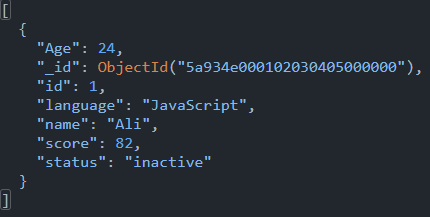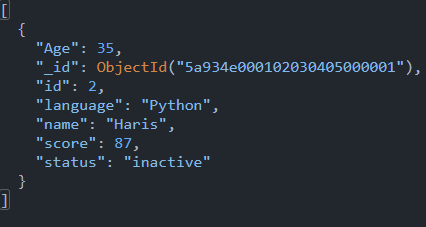# Query With Multiple Conditions in MongoDB

Tahseen Tauseef May 06, 2022

This article will teach you how to query with multiple conditions using multiple documents in MongoDB. `\$and` and `\$or` operators are explained briefly with examples of each.

MongoDB provides the user with different logical query operators, `\$or` and `\$and` operators are one. But first, let’s look at the table of contents for this article.

1. `\$or` operator
2. Find multiple conditions using `\$or` operator
3. `\$and` operator
4. Find multiple conditions using `\$and` operator

## `\$or` Operator in MongoDB

According to the requirements, you can use this operator in methods like `find()`, `update()`, etc.

1. You can also use this operator with text queries, `GeoSpatial` queries, and sort operations.
2. When MongoDB evaluates the clauses in the `\$or` expression, it performs a collection scan. Or, if indexes support all the clauses in the `\$or` expression, then MongoDB performs index scans.
3. You can also nest `\$or` operation.

By executing a logical `OR` operation on an array of two or more `expressions`, the `OR` operator selects documents that satisfy at least one of the `expressions`. The `\$or` operator has the following syntax.

``````{ \$or: [ { <expression1> }, { <expression2> }, ... , { <expressionN> } ] }
``````

Consider the following example.

``````db.inventory.find( { \$or: [ { quantity: { \$lt: 51 } }, { price: 20 } ] } )
``````

In this query, any documents in the inventory collection with a `quantity` field value of less than `51` or the `price` field value of less than `20` will be returned.

Let’s first discuss the behavior of the `or` operator in MongoDB.

### Clauses and Indexes for the `\$or` Operator

When evaluating all the clauses in the `\$or` expression, MongoDB does either a collection scan or an index scan. MongoDB executes index scans if the indexes support all of the clauses.

As a result, for MongoDB to analyze the statement, all clauses in the `\$or` expression must be supported by indexes. If this isn’t the case, MongoDB will do a collection scan.

Each clause of the `\$or` query can utilize its index when employing indexes with `\$or` queries. Look at the following example below.

``````db.inventory.find( { \$or: [ { quantity: { \$lt: 51 } }, { price: 20 } ] } )
``````

Rather than a compound index, you would establish one index for `quantity` and another for the `price` to handle this query.

``````db.inventory.createIndex( { quantity: 1 } )
db.inventory.createIndex( { price: 1 } )
``````

To enable the `\$or` clauses, MongoDB can use any indexes except the `geoHaystack` index.

### `text` Queries and the `\$or` Operator

If `\$or` includes a `\$text` query, an index must support all clauses in the `\$or` array. As a result, a `\$text` query must use an index, and an `\$or` query may only use the indexes if all of its clauses are supported.

If the `\$text` query does not utilize an index, the query will return an error.

### `GeoSpatial` Queries and the `\$or` Operator

`\$or` permits geographic clauses, except for the `near` clause (which includes `\$nearSphere` and `\$near`). There can’t be a relative clause in `\$or` with another clause.

### Sort Operations and the `\$or` Operator

When performing `\$or` queries with a `sort()`, MongoDB may now utilize indexes that support the `\$or` clauses. In prior versions of MongoDB, the indices were not used.

### `\$or` VS `\$in`

Use the `\$in` operator instead of the `\$or` operator with `expressions` that are equality tests for the value of the same field.

Use the `\$in` operator, for example, to pick all documents in the `inventory` collection where the `quantity` field value is either `40` or `1000`.

``````db.inventory.find ( { quantity: { \$in: [40, 1000] } } )
``````

## Find Multiple Conditions Using the `\$or` Operator

The `or` operator in MongoDB is used to select or retrieve only documents that match at least one of the provided phrases.

1. You can also use this operator in a method like a `find()`, `update()`, etc., as per the requirements.
2. You can also use this operator with text queries and sort operations.
3. You can also nest `\$or` operation.

Let’s go for an example to understand the working of the `\$or` operator.

Example:

Let’s create a collection containing the following documents.

``````db={
"students": [
{
id: 1,
name: "Ali",
Age: 24,
language: "JavaScript",
status: "inactive",
score: 82
},
{
id: 2,
name: "Haris",
Age: 35,
language: "Python",
status: "inactive",
score: 87
},
{
id: 3,
team: "Hamza",
Age: 27,
status: "active",
language: "Python",
score: 84
}
]
}
``````

Show all documents from a collection using the `find()` method.

``````db.students.find();
``````

The following is the MongoDB query with the `\$or` operator.

``````db.students.find({
\$or: [
{
Age: {
\$lte: 24
}
},
{
score: {
\$lt: 80
}
},
{
status: "Inactive"
}
]
})
``````

This will produce the following output.## `\$and` Operator in MongoDB

`\$and` perform a logical `AND` operation on an array of one or more expressions (`expression1>`, `expression2>`, etc.) and select the documents that satisfy all of the expressions.

Behavior:

`\$and` handles mistakes as follows to assist the query engine in improving queries.

1. If any expression provided to `\$and` would result in an error if evaluated alone, the `\$and` containing the expression may result in an error, although this is not guaranteed.
2. An expression supplied after the first expression supplied to `\$and` may cause an error even if the first expression evaluates to `false`.

Syntax:

The syntax used when using the `and` operator is the following.

``````{ \$and: [{ <expression1> },{ <expression2> }, ... ,{ <expressionN> }] }
``````

For example, the following query will always produce an error if `\$x` is `0`.

``````db.example.find( {
\$expr: { \$eq: [ { \$divide: [ 1, "\$x" ] }, 3 ] }
} )
``````

The following query, which contains multiple expressions supplied to `\$and`, may produce an error if there is any document where `\$x` is `0`.

``````db.example.find( {
\$and: [
{ x: { \$ne: 0 } },
{ \$expr: { \$eq: [ { \$divide: [ 1, "\$x" ] }, 3 ] } }
]
} )
``````

## Find Multiple Conditions Using the `\$and` Operator

You may use the `\$and` operator in MongoDB to apply several criteria. First, we will choose all papers that fulfill all condition phrases using the `\$and` operation.

1. You can use this operator in methods like `find()`, `update()`, etc., as per the requirement.
2. You can also use this operator with text queries and sort operations.

So let’s understand it with the help of an example.

The following records are inserted into the `students` collection.

``````db={
"students": [
{
id: 1,
name: "Ali",
Age: 24,
language: "JavaScript",
status: "inactive",
score: 82
},
{
id: 2,
name: "Haris",
Age: 35,
language: "Python",
status: "inactive",
score: 87
},
{
id: 3,
team: "Hamza",
Age: 27,
status: "active",
language: "Python",
score: 84
}
]
}
``````

Now, you apply multiple conditions using the `\$and` operator and find the documents.

``````db.students.find({
\$and: [
{
"language": "Python"
},
{
"Age": 35
}
]
})
``````

Here, the `\$and` operation is applied to find those documents where `language` is the `Python`, and `Age` is `35`.

See the working of the above code in MongoDB from this screenshot.You have got the result successfully as per the given condition. Then, you can use the `\$and` operator and retrieve the documents according to the condition.

In this article, querying with multiple conditions using multiple documents in MongoDB is explained in detail using the `\$and` and `\$or` operator.